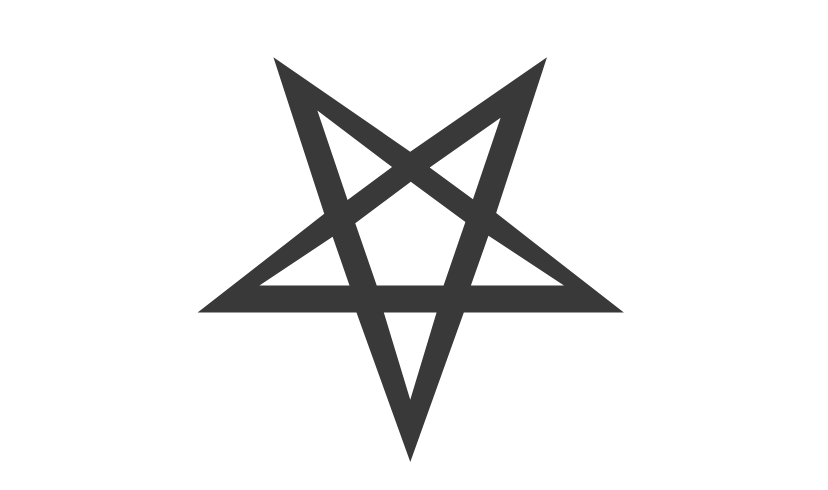# ⛧ Pentagram Copy and Paste

• Symbol copy and pasteAny of the pentagrams you want to copy and paste, just click on the chosen symbol the “Pentagram copy and paste“, ⛤ ⛧ ⛥ ✪ ⍟:

## Pentagram Meaning:

The pentagram is a figure that can be constructed by a single line, a closed interlaced line being considered a symbol of perfection. The pentagram is also known as an infinite loop, as it is possible to make another smaller pentagram within the regular pentagon of the larger pentagram, and so on.

The pentagram is a very old symbol of what one might think. In the West some claim that this symbol was born with Solomon, but it was already used in ancient Egypt where there are records in tombs and sarcophagi.

The pentagram is also found in Chinese culture representing the cycle of destruction, which is the philosophical basis of their traditional medicine. In this case, each end of the pentagram symbolizes a specific element: Earth, Water, Fire, Wood and Metal.

Pythagoras discovered that this symbol had some interesting properties. A pentagram is obtained by drawing the diagonals of a regular pentagon; by the intersections of the segments of this diagonal, a new regular pentagon is obtained, which is proportional to the original exactly by the golden ratio.

If we join one end of the star with the two opposite ones, it forms an isosceles triangle. This triangle has two angles of 72° and a third of 36°, so half of each of the larger ones. Such a polygon is called a golden triangle. Interestingly, if we bisect one of the larger angles by splitting the original triangle in two, the resulting smaller triangle looks similar to the original, that is, it is again a golden triangle. By dividing this triangle by the same process, an infinite succession of nested golden triangles can be constructed.

Another curious geometric succession can be constructed by noting that the points of the pentagram draw a regular pentagon that surrounds the star. Looking inside, we again discover a regular pentagon. This means that you can build an infinite succession of nested pentagons and pentagrams.

http://www.projetos.unijui.edu.br/matematica/cd_egem/fscommand/CC/CC_21.pdf